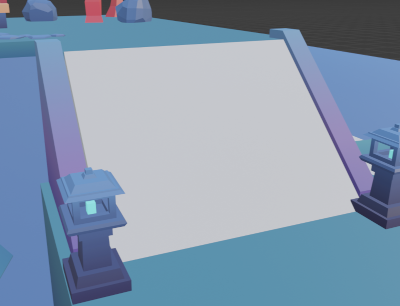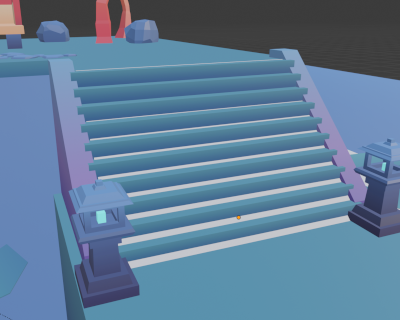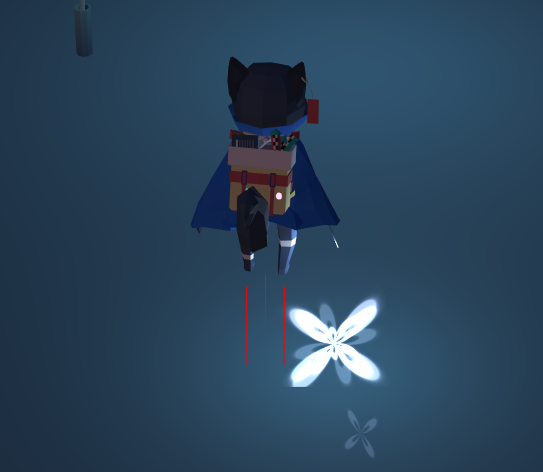# Character Movement Part 2

Now that we have our character moving around, let's give them the ability to navigate platforms!

## Input Controller

First we'll start by adding in inputs for our jumping and dashing to inputController.ts! Inside of updateFromKeyboard we just need to add:

``````//dash
if (this.inputMap["Shift"]) {
this.dashing = true;
} else {
this.dashing = false;
}

//Jump Checks (SPACE)
if (this.inputMap[" "]) {
this.jumpKeyDown = true;
} else {
this.jumpKeyDown = false;
}
``````

this.dashing and this.jumpKeyDown will be used to control the actions based off of conditions being met in characterController.ts.

## Jumping

Here we'll be focusing on _updateFromGroundDetection. We'll be adding and updating what we wrote in part 1's gravity.

### Input

``````//Jump detection
if (this._input.jumpKeyDown && this._jumpCount > 0) {
this._gravity.y = Player.JUMP_FORCE;
this._jumpCount--;
}
``````

Here we're checking whether our jumpKeyDown is true and the jumpCount is > 0. If these are true, then we add to our gravity a jump force (which is defined as a negative number) to propel a force upwards so that the character jumps.

### Grounded Check

``````if (this._isGrounded()) {
this._gravity.y = 0;
this._grounded = true;
this._lastGroundPos.copyFrom(this.mesh.position);

this._jumpCount = 1; //allow for jumping
}
``````

We want to reset the jumpCount back to 1. This is where you could potentially allow for double jumps, but I chose to stick to a single jump mechanic.

## Slopes

I specifically added the implementation for detecting slopes because I wanted there to be stairs in the game. This served to be a challenge because meshes use their actual geometry, so in order to accomplish a smooth walking movement along stairs, I needed to create invisible colliders. These were just box meshes that were rotated to have the flat side along where the stairs would be (essentially a slope).Then I had to differentiate between stairs and non-stairs. The way I did this was by comparing the angles between the normal of the mesh and the up vector (this took some testing to find a good range).

The _checkSlope function can be broken down as follows:

1. The predicate is a function that defines what types of meshes are eligible for being picked by a raycast. We define the mesh that we're looking for as being pickable and enabled since we want to include meshes that are not visible.
``````let predicate = function (mesh) {
return mesh.isPickable && mesh.isEnabled();
}
``````
2. We send 4 raycasts down from the character, making a kind of circle of raycasts.3. For each raycast, we're checking if there's a hit and whether the normal of the hit is not equal to the up vector.
``````if (pick.hit && !pick.getNormal().equals(Vector3.Up())) {
//check whether it's stairs or not
}
``````
4. I implemented this calculation for stairs in two ways:
• Originally, I calculated the angle between the normal of the picked mesh and the up vector and if the angle was within a range, it was considered a slope.
``````let dot = Vector3.Dot(pick.getNormal(), Vector3.Up());
let angle = Math.acos(dot / (pick.getNormal().length() * Vector3.Up().length()));
return angle > 0.70 && angle < 1.6;
``````
• However, because there were some other meshes whose normals matched that range, it messed with the character animations in some areas as it detected them as slopes. So, I instead just checked to see if the pickedMesh's name had "stair" in it
``````if(pick.pickedMesh.name.includes("stair")) {
return true;
}
``````
This range may be hardcoded and based specifically on the meshes used, so there's definitely a better way to do this. One potential way is to use tags to signify the specific meshes that are stairs and check for whether the raycasts are hitting those.

Now, we want to update our if not grounded check to take into account slopes:

``````if (!this._isGrounded()) {
//if the body isnt grounded, check if it's on a slope and was either falling or walking onto it
if (this._checkSlope() && this._gravity.y <= 0) {
//if you are considered on a slope, you're able to jump and gravity wont affect you
this._gravity.y = 0;
this._jumpCount = 1;
this._grounded = true;
} else {
//keep applying gravity
this._grounded = false;
}
}
``````

We are checking for slopes here because as the character walks along the slope, they're actually considered not grounded based off of the single center raycast that we're doing in the _isGrounded function. Thus, the player has gravity continuously acting on them, pushing them down the slope.

This is why we check for whether we're on a slope, and then remove gravity, and fake being grounded. We also only check if our gravity tells us that we're walking up the stairs and not down, so essentially as our character "walks" down the stairs, gravity is acting on them (kind of like in real life!)

## Dashing

Dashing was another mechanic that I thought would be useful for a platformer. Since this game is based on time, I wanted to add a limitation on the dash mechanic so that it wouldn't become the main mode of transportation. Thus, the dash move became an air-dash that's limited to being used once until you touch a ground again.

### Input

``````if (this._input.dashing && !this._dashPressed && this._canDash && !this._grounded) {
this._canDash = false; //we've started a dash, do not allow another
this._dashPressed = true; //start the dash sequence
}
``````

This seems like a lot of conditions, and that's because we're limiting the dash to a single instance if we're in the air.

### Duration

``````let dashFactor = 1;
//if you're dashing, scale movement
if (this._dashPressed) {
if (this.dashTime > Player.DASH_TIME) {
this.dashTime = 0;
this._dashPressed = false;
} else {
dashFactor = Player.DASH_FACTOR;
}
this.dashTime++;
}
``````

If we've begun our dash sequence, we want to increment our timer every frame. If we are in the dashing sequence, we set our dashFactor. If we've completed our dash, we reset our timer and set _dashPressed to false to exit the sequence.

### Reset

Returning to the grounded check, this is where we want to reset our dashing ability.

``````//dashing reset
this._canDash = true; //the ability to dash
//reset sequence(needed if we collide with the ground BEFORE actually completing the dash duration)
this.dashTime = 0;
this._dashPressed = false;
``````

### Modifying Movement

We want to update _updateFromControls to now take into account our dashing.

``````//clear y so that the character doesnt fly up, normalize for next step, taking into account whether we've DASHED or not
this._moveDirection = new Vector3((move).normalize().x * dashFactor, 0, (move).normalize().z * dashFactor);
``````

Previously where we just normalized our move vector, we want to multiply by the dashFactor to give it that extra bit of movement in the direction we're currently moving.

Now, if we run the game, we should be able to move, jump, and dash! You will notice that we still have that issue of falling into the ground. I am not sure why this is happening, but it doesn't happen once you import the final mesh. However, early on in the development I had fixed this issue before re-writing the gravity & jumping code. You can take a look at what I did here. Essentially, We have re-adjust to account for how much we would fall through. In addition, having more raycasts that are longer will help in detecting the ground earlier.